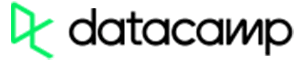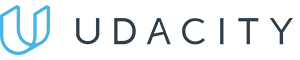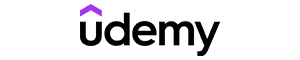Code has been added to clipboard!

# All Types of the Python Queue Explained

Published Feb 19, 2020
Updated Feb 19, 2020

TL;DR – A Python queue is a linear data structure, similar to a stack.

## How to use a queue in Python

To start building Python queues, you need to import the `queue` Python module first:

``import queue``

Python 1.4 and all the newer versions have this module available for use. It allows you to implement Python multithreading queues:

• To add an element to the queue, use `put()`. This is called an enqueue operation.
• To remove an element from the queue, use `get()`. This is called a dequeue operation.
• The FIFO (First In, First Out) principle means the first element you insert will also be the first to be removed.

By using the `maxsize` argument, you can choose to restrict the number of elements in a queue. To leave the size unlimited, define it as `0` or a negative number.Pros
• Easy to use with a learn-by-doing approach
• Offers quality content
• Gamified in-browser coding experience
• The price matches the quality
• Suitable for learners ranging from beginner to advanced
Main Features
• Free certificates of completion
• Focused on data science skills
• Flexible learning timetablePros
• Simplistic design (no unnecessary information)
• High-quality courses (even the free ones)
• Variety of features
Main Features
• Nanodegree programs
• Suitable for enterprises
• Paid Certificates of completionPros
• Easy to navigate
• No technical issues
• Seems to care about its users
Main Features
• Huge variety of courses
• 30-day refund policy
• Free certificates of completion

## Python queue examples: four types

To create a basic Python queue, use `queue.Queue()`:

Example
``````BitDegree = queue.Queue(maxsize=0)

BitDegree.put("B")
BitDegree.put("i")
BitDegree.put("t")

print (BitDegree.get())``````

You can also create a queue in Python that follows the LIFO principle (Last In, First Out). In such a case, the first element to remove will be the one that got added last. To do that, use `queue.LifoQueue()`:

Example
``````import queue

BitDegree = queue.LifoQueue(maxsize=0)

BitDegree.put("B")
BitDegree.put("i")
BitDegree.put("t")

print (BitDegree.get())``````

If you need to sort your elements in a specific way, you can also create a priority queue. For that, you would use `queue.PriorityQueue()`. In such a case, the first element to remove will be the one that has the lowest value:

Example
``````BitDegree = queue.PriorityQueue(maxsize=0)

BitDegree.put((3, "B"))
BitDegree.put((1, "i"))
BitDegree.put((2, "t"))

print (BitDegree.get())``````

If you're using Python 3.7 or a newer version, you can also use `queue.SimpleQueue()` to create simple Python queues with less functionalities – e.g, they will not allow task tracking:

Example
``````BitDegree = queue.SimpleQueue(maxsize=0)

BitDegree.put("B")
BitDegree.put("i")
BitDegree.put("t")``````

## Python queue: useful tips

• To simplify working with priority queues, follow the `number, element` pattern and use the `number` to define priority.
• To create Python multiprocessing queues (as opposed to multithreading), use `multiprocessing.Queue()` function for the `multiprocessing` module.

Days
Hours
Minutes
Seconds